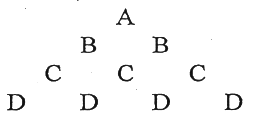MORE IN Structured Programming Approach
MU First Year Engineering (Semester 2)
Structured Programming Approach
December 2014
Total marks: --
Total time: --
INSTRUCTIONS
(1) Assume appropriate data and state your reasons
(2) Marks are given to the right of every question
(3) Draw neat diagrams wherever necessary

1 (a) Explain the difference in using call by reference and call by value methods for argument passing, for swapping 2 numbers.
8 M
1 (b) What is significance of storage class? Illustrate each storage class with example.
10 M
1 (c) Find the output of the following code:
main ()
{
int a[]={10,20,30,40,50};
int *j, *k;
j=&a;
k=(a+4);
if (j==k)
printf ("Both pointers points to same locations");
else
printf ("both pointers does not point to same locations");
}
2 M

2 (a) Choose the correct answer for the following:
(i) Parameters passed as arguments to the function call are called as
(a) Actual parameters
(b) No parameters
(c) Formal parameters
(d) None of these

(ii) Which of the following returns statement in function has error
(a) return
(b) return (expression)
(c) return (0)
(d) None of these

(iii) Which of the following is not jump statement
(a) for
(b) continue
(c) break
(d) goto

(iv) Which of the following is an exit controlled loop.
(a) for
(b) do-while
(c) while
(d) if-else

(v) In array a={10,20,30,40,50} then a element is
(a) 10
(b) 40
(c) 50
(d) 20
5 M
2 (b) Write a program using function to print first 'n' numbers in Fibonacci series.
5 M
2 (c) Define structure within structure consisting of following elements :-
(i) Employee code
(ii) Employee name
(iii) Employee salary
(iv) Employee date of joining.
Write a program to read at least 10 records and display them.
10 M

3 (a) Write a program to implement calculator with following operations:-
(ii) Division two numbers
(iii) Subtract two numbers
(iv) Multiply two numbers
6 M
3 (b) Write a program to find the GCD and LCM of 2 nos.
6 M
3 (c)

Write a program to print the following pattern. (Note: Not only 4 lines, it should print n line taken from user)8 M

4 (a) Write a program to find the biggest of given 3 no's using conditional operator.
5 M
4 (b) Write a program to check whether entered string is palindrome or not.
5 M
4 (c) Write a program in C to read and display elements of a square (2D) matrix and check whether the matrix is symmetric or not.
10 M

5 (a) Evaluate following expression:-
(i) !(5+5<10)
(ii) 5+5==10 || 1+3==5
(iii) 5.10|| 10<20 && 3<5
3 M
5 (b) An electric power distribution company charges its domestic consumer as follows:
 Consumption Units Rate of Charge 0-200 0-50 per unit 201-400 Rs. 100 plus Rs. 0.65 per unit excess of 200 401-600 Rs. 230 plus Rs. 085 per unit excess of 400 601- above Rs. 390 plus Rs. 1.00 per unit excess of 600

Program should read the units consumed for a customer and calculate the total bill.
7 M
5 (c) Four experiments are performed, each experiment consisting of six test results. The result for each experiment follows. WAP to compute and display the average of the test results for each experiment.
 1st Experiment results 23.2 31.5 16.9 28 26.3 28.2 2nd Experiment results 34.8 45.2 20.8 39.4 33.4 36.8 3rd Experiment results 19.4 50.6 45.1 20.8 50.6 13.4 4th Experiment results 36.9 42.7 20.8 10.2 16.8 42.7
10 M

6 (a) Explain with example bitwise operators in C language.
5 M
6 (b) Write a program using recursive function 'power' to compare xn
 power (x,n)=1 if n=0 power (x,n)=x if n=1 power (x,n)=x*power(x, n-1) otherwise
8 M
6 (c) Write a user defined function to copy one string to another.
4 M
6 (d) What is a File? What are the different mode in which we can open a file? What are the different functions to read and write to file?
3 M

More question papers from Structured Programming Approach